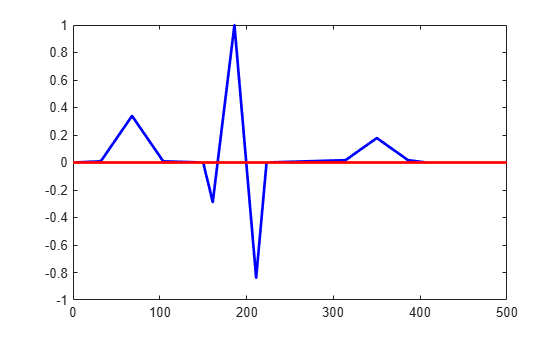dsp.ZeroCrossingDetector

Detect zero crossings

Description

The dsp.ZeroCrossingDetector System object™ counts the number of times the signal crosses zero, or changes sign. To detect if a signal in a given channel crosses zero, the object looks for the following conditions, where, xi-l is the current signal value and xi-1 is the previous signal value:

• xi < 0 and xi-1 > 0

• xi > 0 and xi-1 < 0

• For some positive integer L, xi < 0, xi-l = 0, and xi-L-1 > 0, where $0\le l\le L$.

• For some positive integer L, xi > 0, xi-l = 0, and xi-L-1 < 0, where $0\le l\le L$.

For the first input value, xi-1 and xi-2 are zero.

To count the number of times a signal crosses zero or changes sign:

1. Create the dsp.ZeroCrossingDetector object.

2. Call the object with arguments, as if it were a function.

Creation

Description

example

zcd = dsp.ZeroCrossingDetector returns a zero crossing detection object that calculates the number of times the signal crosses zero.

Usage

Description

example

zcdOut = zcd(input) calculates the number of zero crossings of the input. Each column of the input is treated as an independent channel.

Input Arguments

expand all

Data input whose zero crossings are counted by the object, specified as a vector or a matrix.

Example: rand(20,1)-0.3

Example: rand(20,2)-0.3

Data Types: single | double | int8 | int16 | int32 | int64 | uint8 | uint16 | uint32 | uint64 | fi

Output Arguments

expand all

Number of zero crossings in the data input, returned as one of the following:

• scalar –– When the data input is a column vector, the scalar output is the number of zero crossings in the data input..

• row vector –– When the data input is a matrix, each element in the row vector output is the number of zero crossings in the corresponding column of the data input.

Example: 10

Example: [9,6]

Data Types: uint32

Object Functions

To use an object function, specify the System object as the first input argument. For example, to release system resources of a System object named obj, use this syntax:

release(obj)

expand all

 step Run System object algorithm release Release resources and allow changes to System object property values and input characteristics reset Reset internal states of System object

Examples

collapse all

Note: If you are using R2016a or an earlier release, replace each call to the object with the equivalent step syntax. For example, obj(x) becomes step(obj,x).

Find the number of zero crossings in electrocardiogram data.

EcgData = ecg(500)';
zcd = dsp.ZeroCrossingDetector;
numZeroCross = zcd(EcgData)
numZeroCross = uint32
4
plot(1:500,EcgData,'b',[0 500],[0 0],'r','linewidth',2)Extended Capabilities